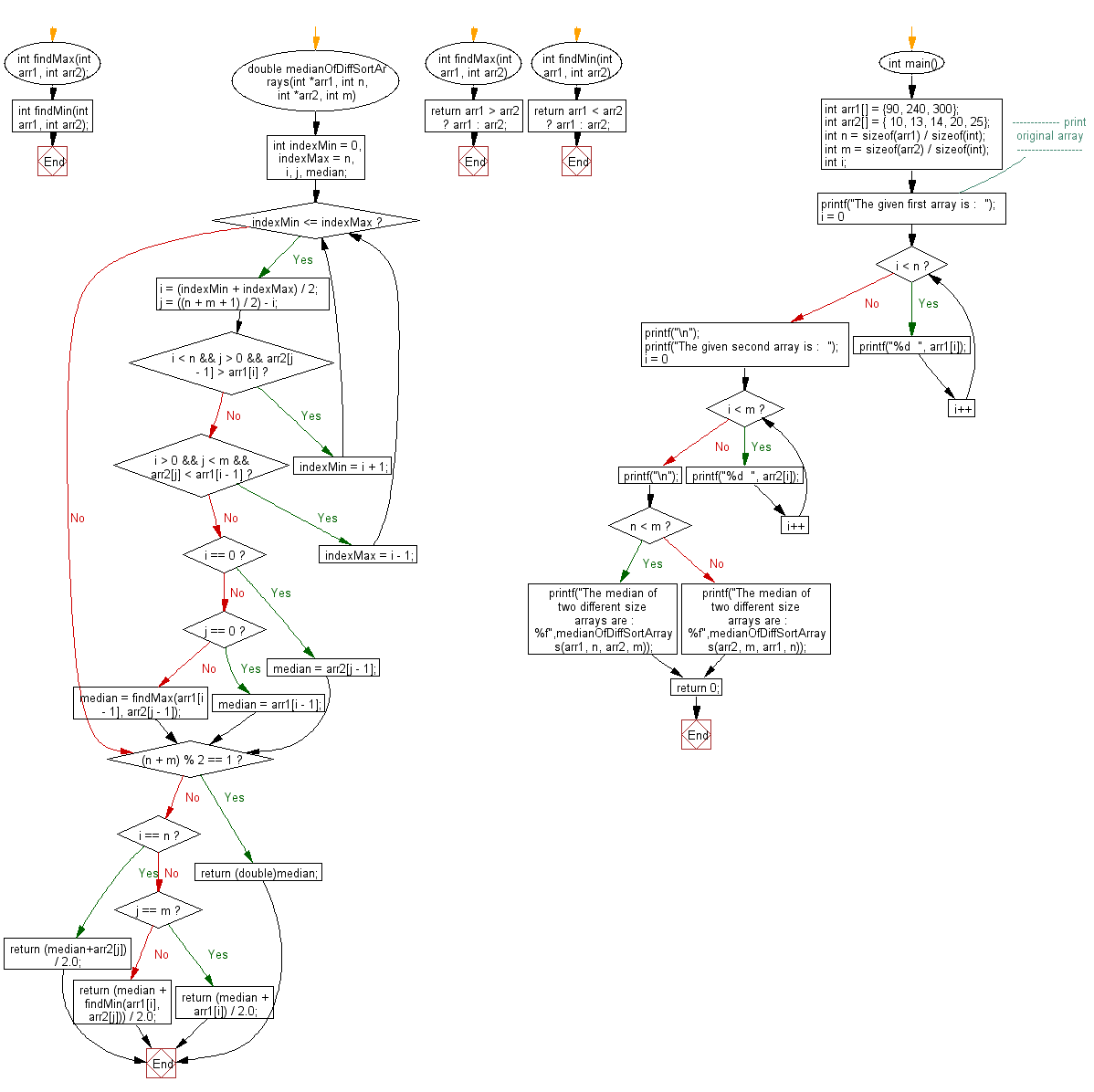﻿ C exercises: Find the median of two sorted arrays of different size - w3resource# C Exercises: Find the median of two sorted arrays of different size

## C Array: Exercise-71 with Solution

Write a program in C to find the median of two sorted arrays of different size.

Sample Solution:

C Code:

``````#include <stdio.h>
int findMax(int arr1, int arr2);
int findMin(int arr1, int arr2);

double medianOfDiffSortArrays(int *arr1, int n,
int *arr2, int m)
{

int indexMin  = 0, indexMax  = n, i, j, median;
while (indexMin <= indexMax)
{
i = (indexMin + indexMax) / 2;
j = ((n + m + 1) / 2) - i;
if (i < n && j > 0 && arr2[j - 1] > arr1[i])
indexMin = i + 1;
else if (i > 0 && j < m && arr2[j] < arr1[i - 1])
indexMax = i - 1;
else
{
if (i == 0)
median = arr2[j - 1];
else if (j == 0)
median = arr1[i - 1];
else
median = findMax(arr1[i - 1], arr2[j - 1]);
break;
}
}
if ((n + m) % 2 == 1)
return (double)median;
if (i == n)
return (median+arr2[j]) / 2.0;
if (j == m)
return (median + arr1[i]) / 2.0;
return (median + findMin(arr1[i], arr2[j])) / 2.0;
}
int findMax(int arr1, int arr2)
{
return arr1 > arr2 ? arr1 : arr2;
}
int findMin(int arr1, int arr2)
{
return arr1 < arr2 ? arr1 : arr2;
}
int main()
{
int arr1[] = {90, 240, 300};
int arr2[] = { 10, 13, 14, 20, 25};
int n = sizeof(arr1) / sizeof(int);
int m = sizeof(arr2) / sizeof(int);
int i;
//------------- print original array ------------------
printf("The given first array is :  ");
for(i = 0; i < n; i++)
{
printf("%d  ", arr1[i]);
}
printf("\n");
printf("The given second array is :  ");
for(i = 0; i < m; i++)
{
printf("%d  ", arr2[i]);
}
printf("\n");
//------------------------------------------------------
if (n < m)
printf("The median of two different size arrays are : %f",medianOfDiffSortArrays(arr1, n, arr2, m));
else
printf("The median of two different size arrays are : %f",medianOfDiffSortArrays(arr2, m, arr1, n));
return 0;
}
```
```

Sample Output:

```The given first array is :  90  240  300
The given second array is :  10  13  14  20  25
The median of two different size arrays are : 22.500000
```

Flowchart:C Programming Code Editor:

Improve this sample solution and post your code through Disqus.

What is the difficulty level of this exercise?

﻿